Conditional mutual information 条件互信息

http://blog.csdn.net/pipisorry/article/details/51695283

• 首先理解信息（I）的定义
I 是衡量信息w的量，只和w发生的概率P(w)有关，认为

I(w)=f(P(w))

并且满足公式
I(A,B)=I(A)+I(B)P(A,B)=P(A)P(B)

若A,B是独立事件，那么AB同时发生的信息量是AB分别发生的信息量之和，而概率则是求乘积。
那么满足公式的加法和乘法条件的f(.)$f(.)$就是log(.)$log(.)$函数了。
所以定义I(w)=log(P(w))$I(w)=-log(P(w))$
详细展开：
https://en.wikipedia.org/wiki/Self-information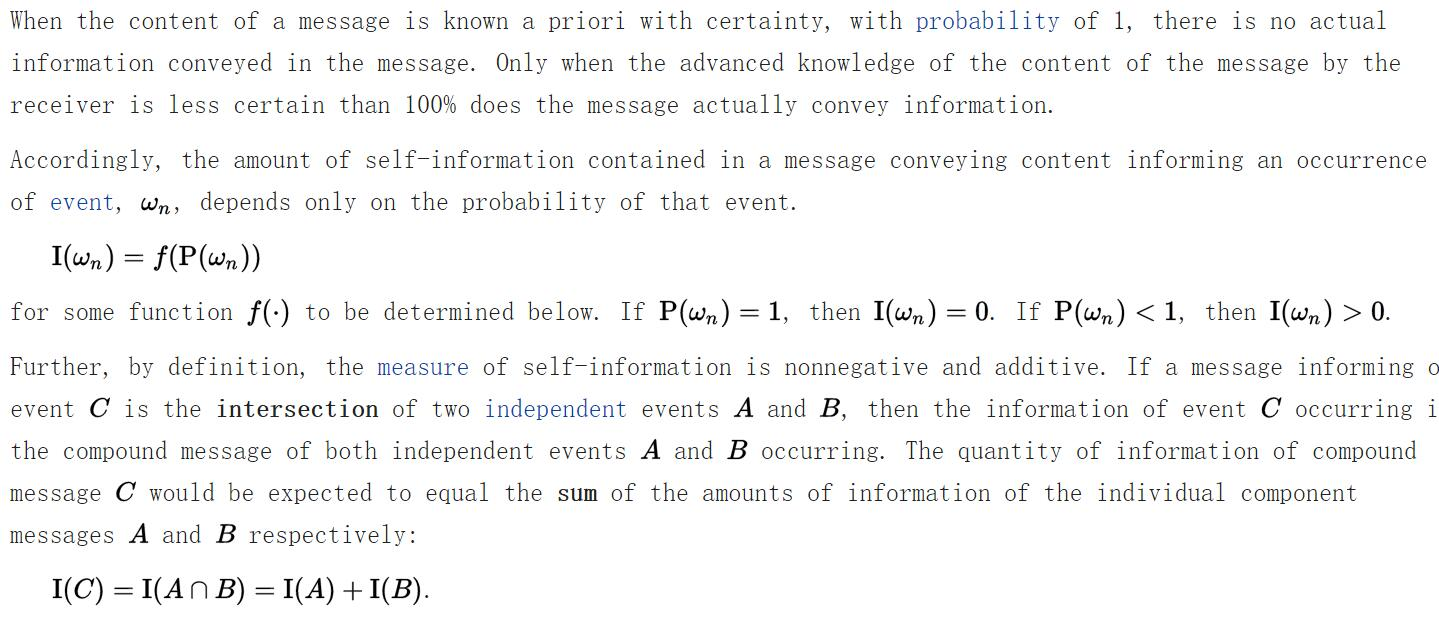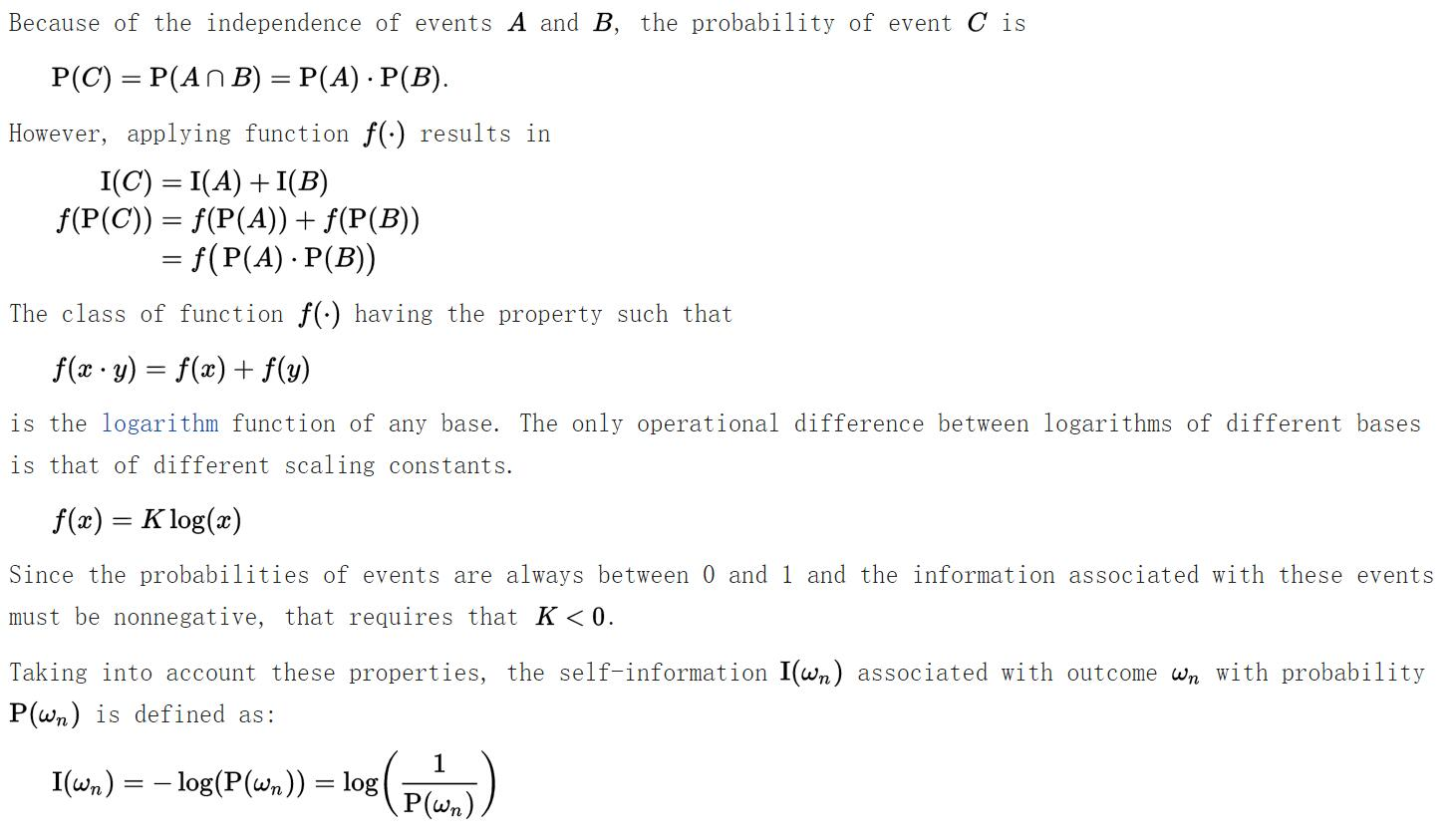• 熵Entropy（E）的定义
熵就是信息的期望，所以要做一个E(w)=P(w)I(w)=P(w)log(P(w))$E(w)=P(w)*I(w)=-P(w)*log(P(w))$
注意到当P(w)等于0时，由于在P(w)极限逼近0时，P(w)log(P(w))等于0，所以规定

if:P(w)=0,then:P(w)log(P(w))=0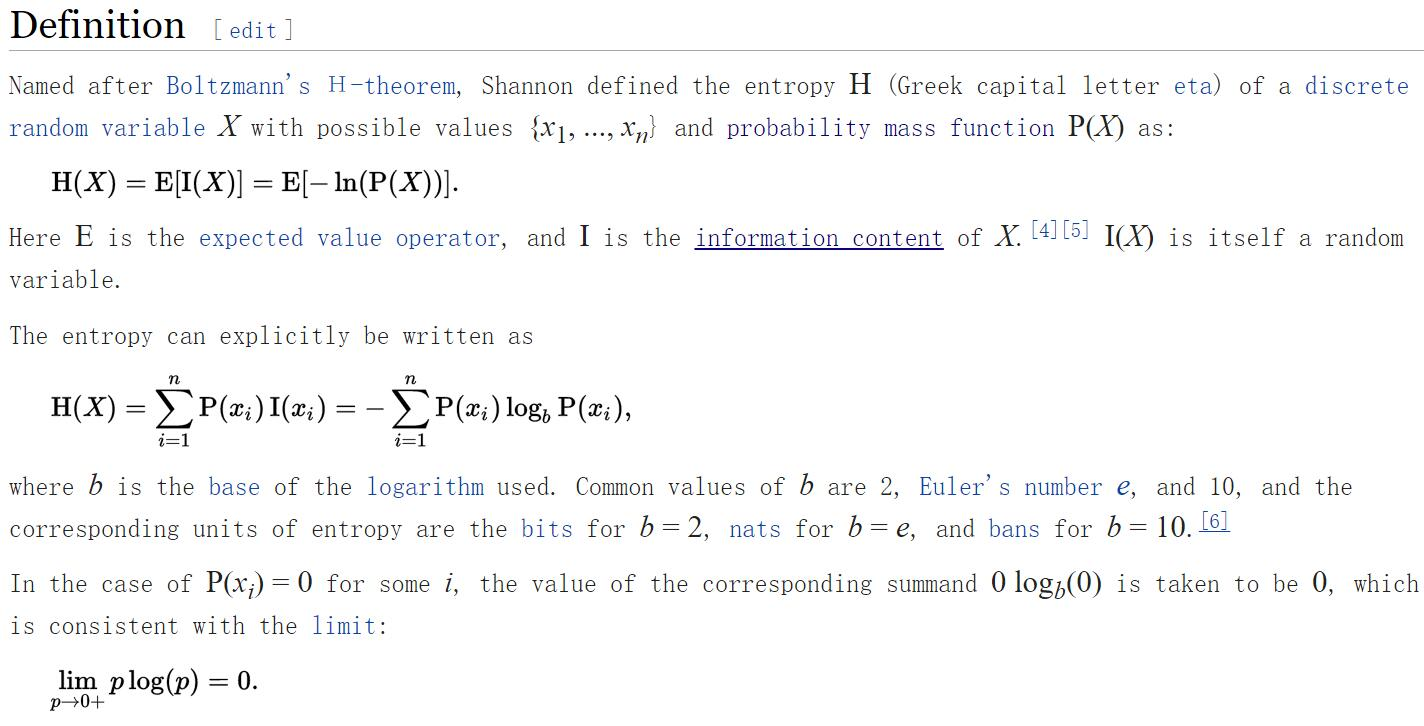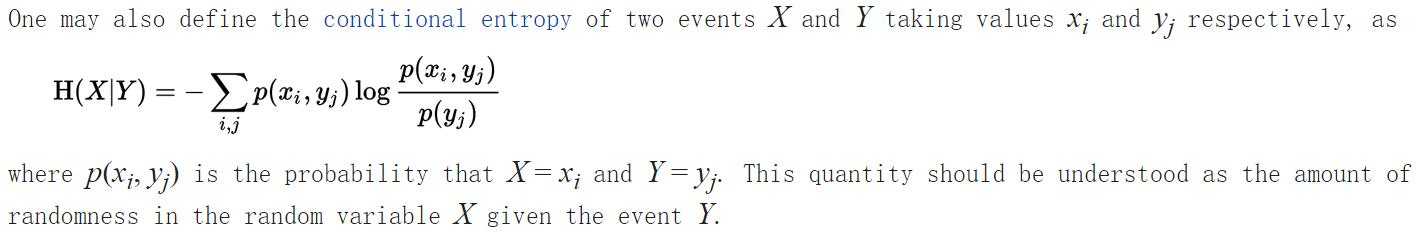• joint entropy 联合熵
描述一个变量集（这个集合包括X,Y）的不确定性
注意H(X,Y)理解为描述X和Y要用到的信息量，那么H(X,Y)包括要来描述X的H(X)+已知X要额外描述Y需要增加的H(Y|X)。而I(X;Y)可以理解为描述X和Y信息关联程度的量。
换到图上，H(X,Y)是两个圆覆盖的总面积，I(X,Y)是重叠面积，H(X|Y)和H(Y|X)是两个圆互不交的地方。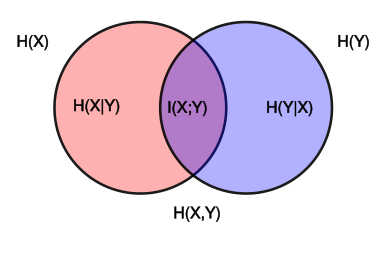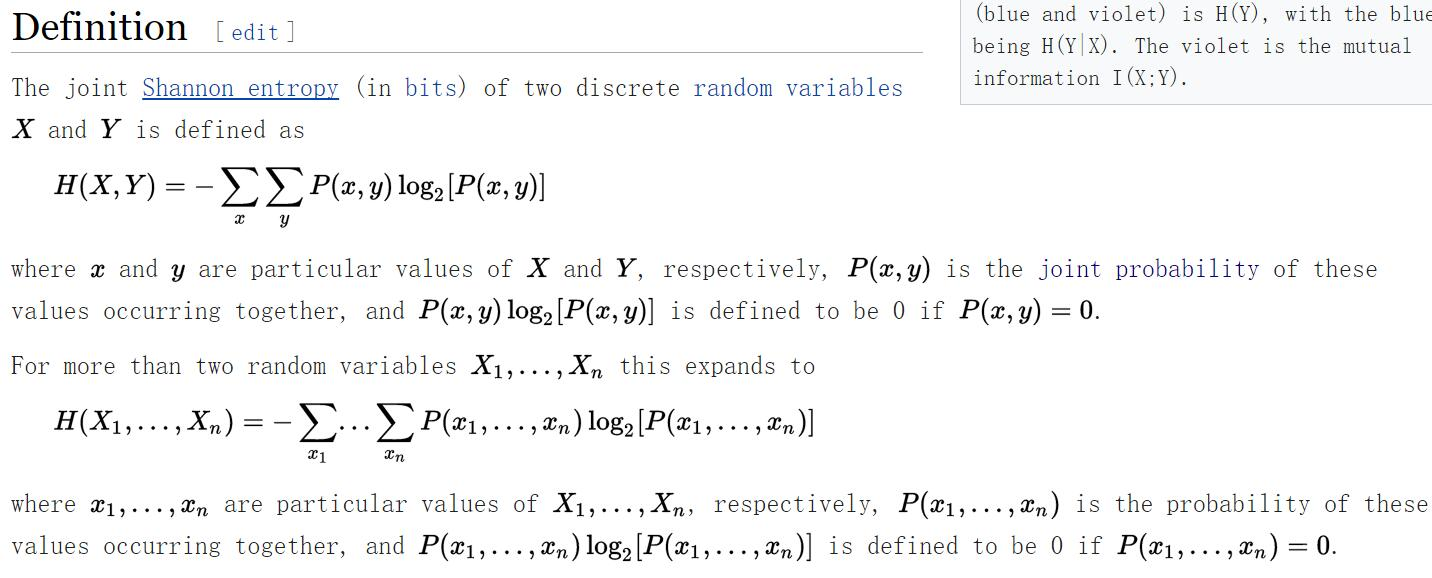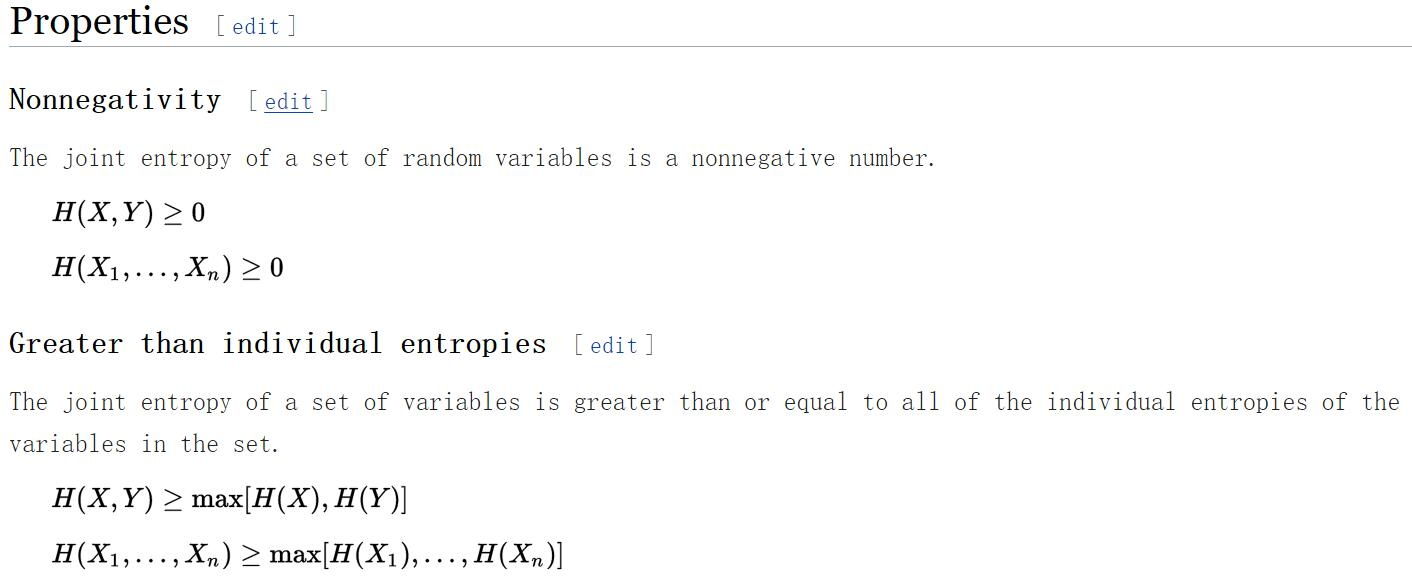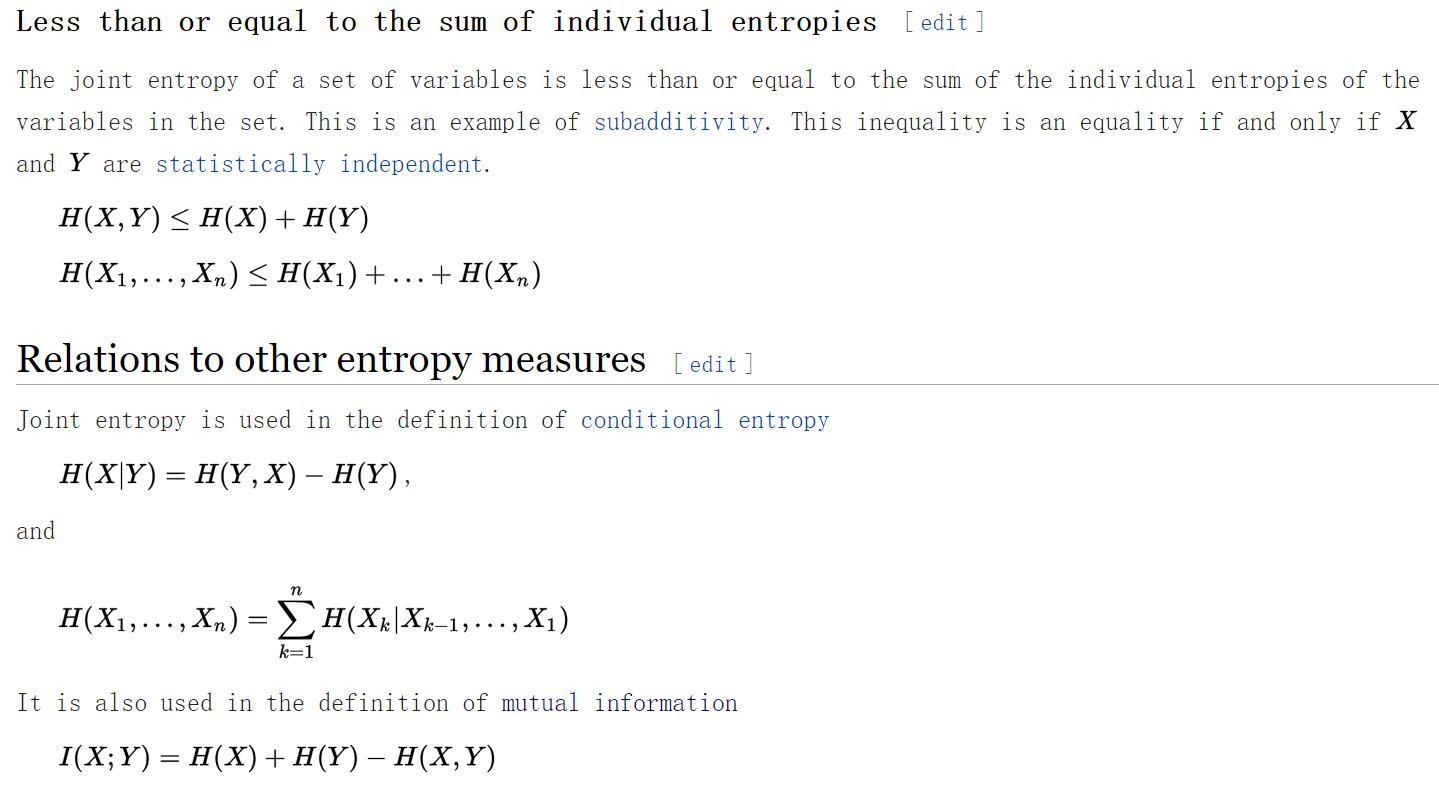• mutul information 互信息
描述X和Y的之间的依赖程度。如果X和Y完全独立，那么p(x,y)=p(x)p(y)$p(x,y)=p(x)p(y)$,则log(1)=0,最后的I(X;Y)=0，如果存在依赖，那么I能够描述出依赖的程度；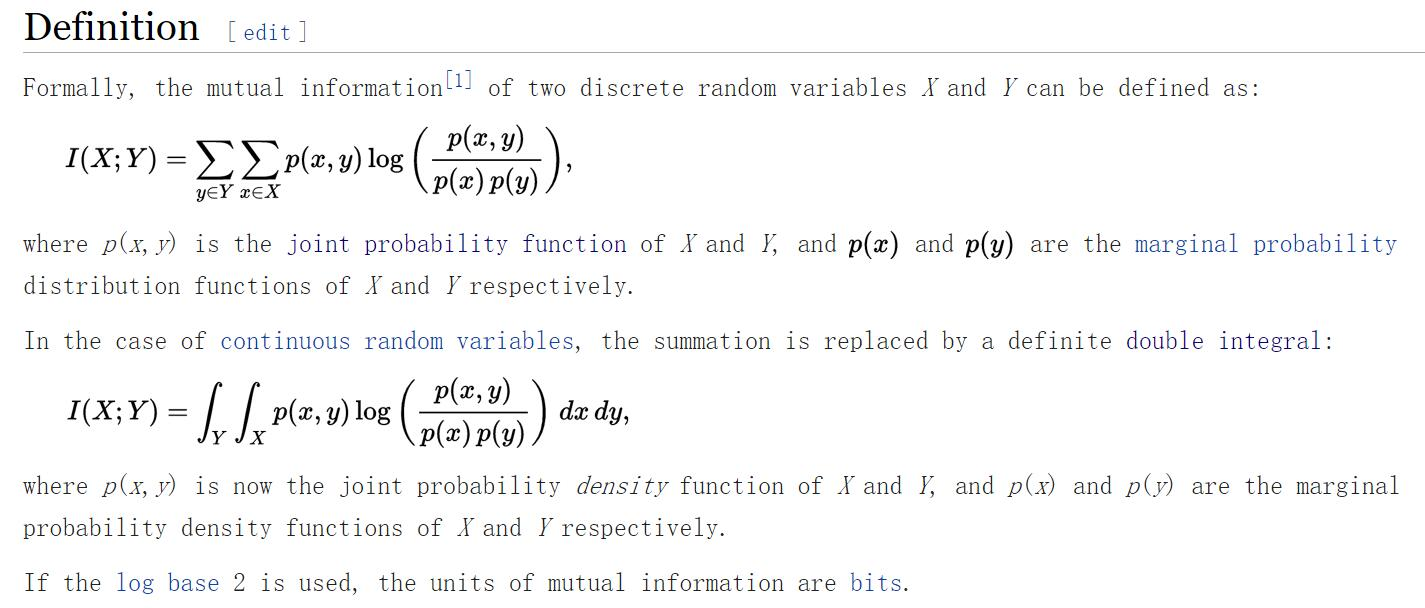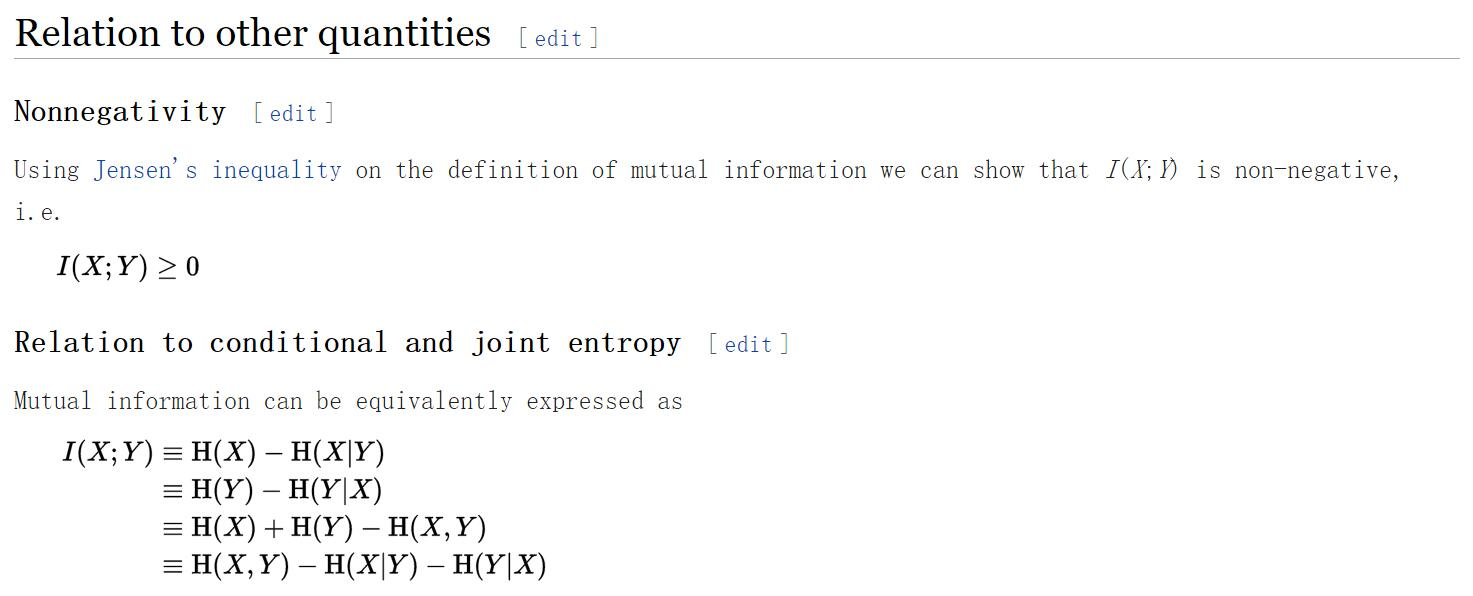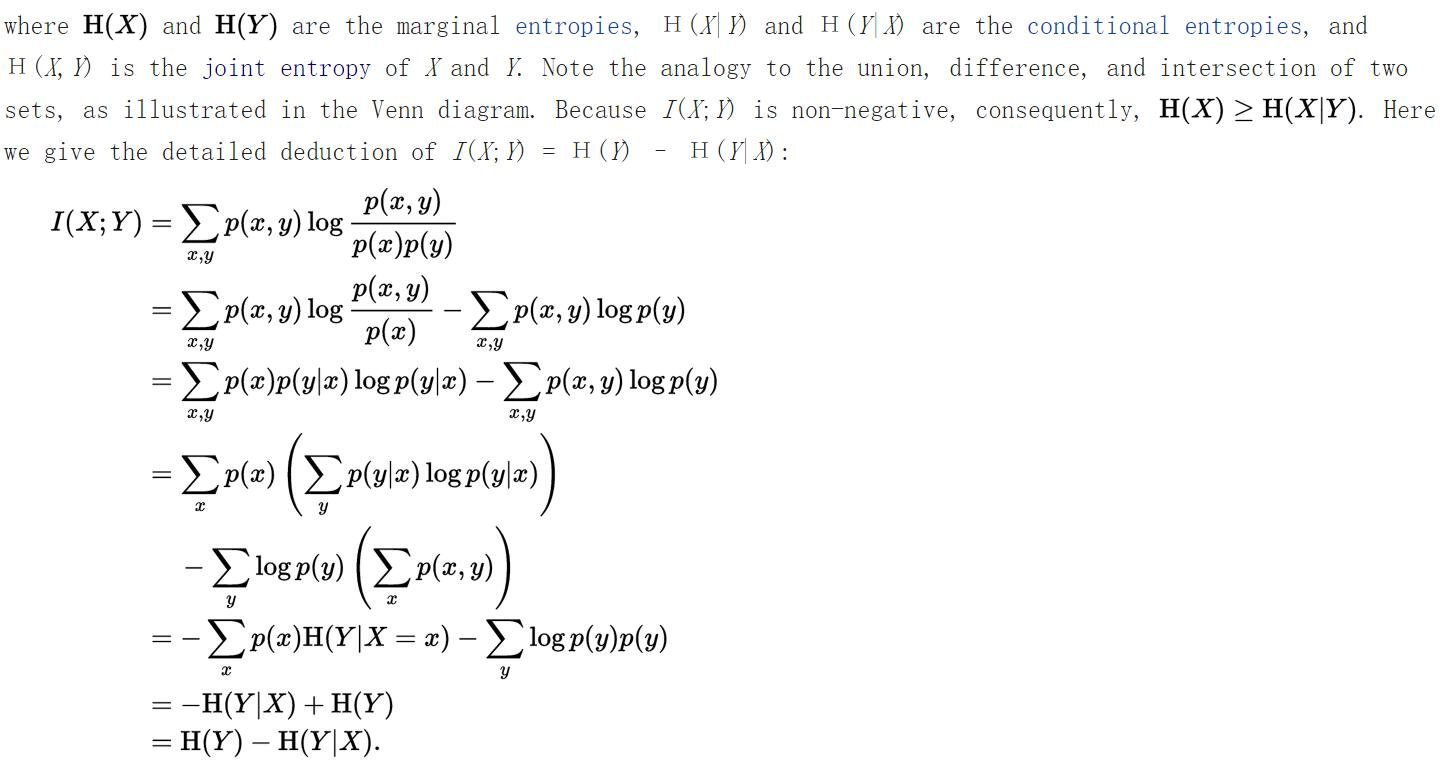• 条件互信息
在条件（Z）发生时的条件互信息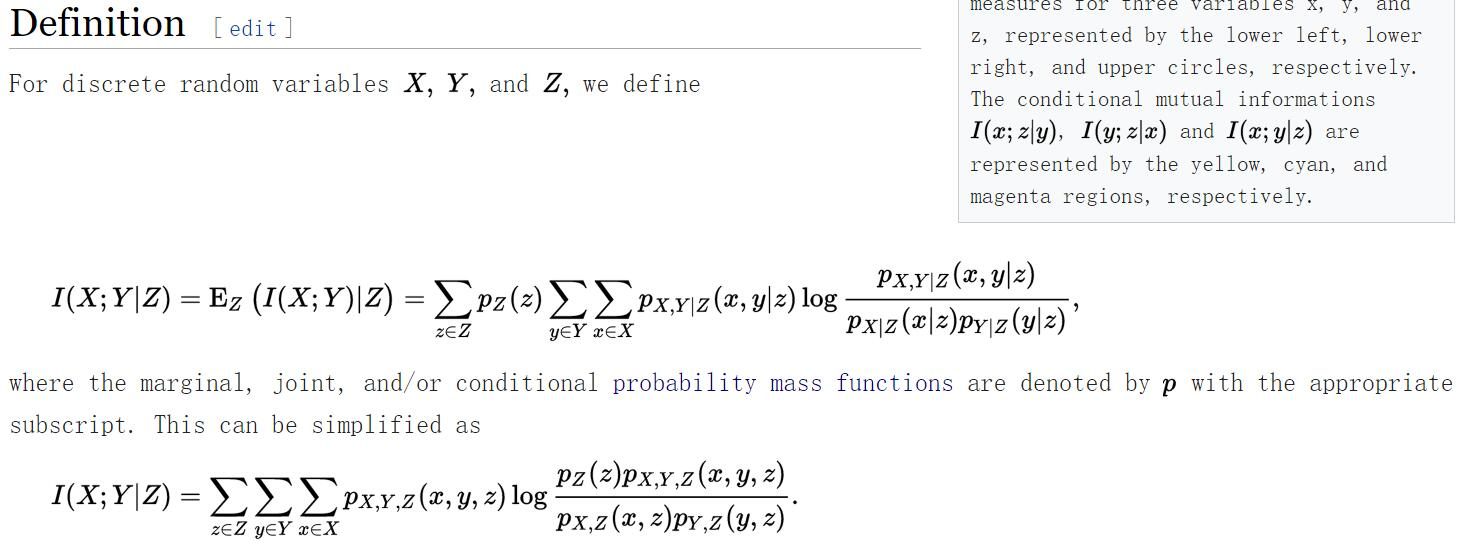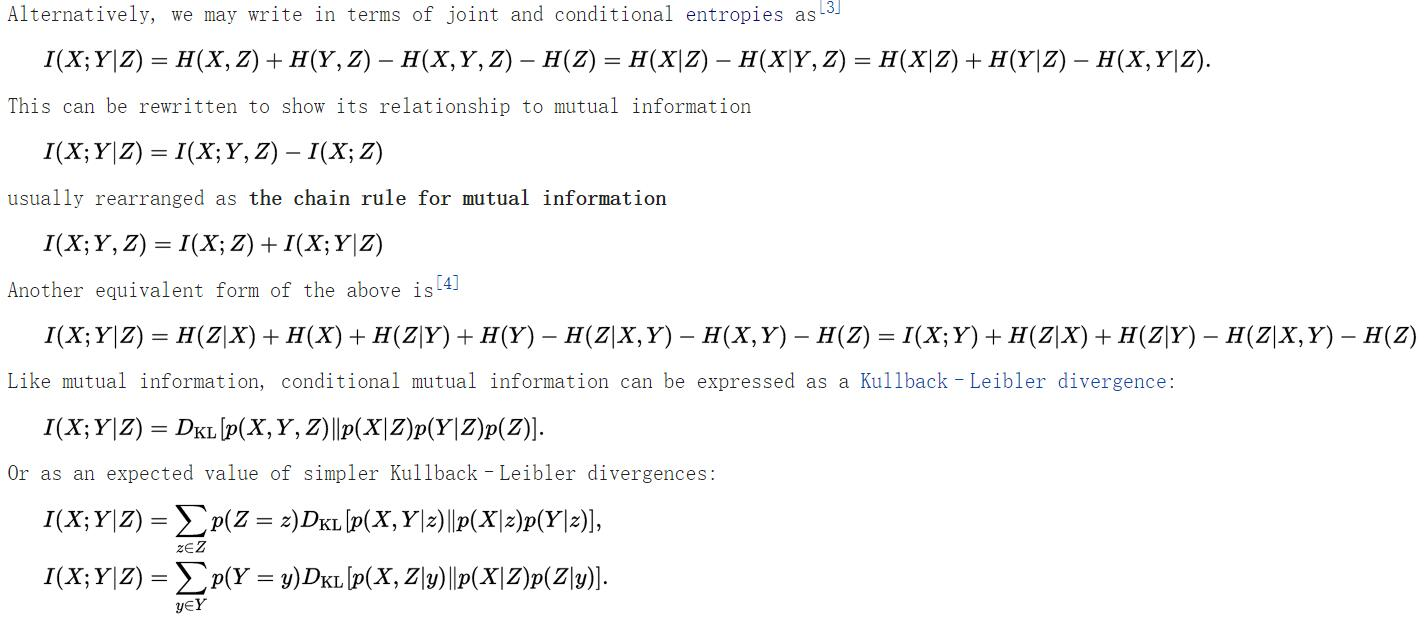总结

• 信息I(w)=f(P(I(w)))
• 信息发生的概率P(I(w))
• 信息*概率=熵(其实就是信息的期望)
• f(.)=Klog(.)
• 优美的加法(I(w)之间)乘法(P(I(w))之间)，他们的联系就是log,是log让他们直接加法变成了乘法。